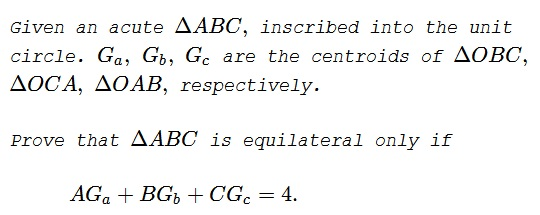# Equilateral Triangle from Three Centroids

### Problem### Solution

Using complex numbers, let $A=a,$ $B=b,$ $C=c,$ with $|a|=|b|=|c|=1.$ Since the circumcenter is at $0,$ we have $\displaystyle G_a=\frac{b+c}{3},$ $\displaystyle G_b=\frac{c+a}{3},$ $\displaystyle G_c=\frac{a+b}{3}.$ Also, the orthocenter $H=a+b+c.$ It follows that

$\displaystyle AG_a=\frac{|3a-b-c|}{3}\le\frac{|3a|+|b+c|}{3}=1+\frac{|b+c|}{3}=1+\frac{AH}{3}.$

Similarly, $\displaystyle BG_b\le 1+\frac{BH}{3}$ and $\displaystyle CG_c=1+\frac{CH}{3}.$ Summing up and taking into the account $AG_a+BG_b+CG_c=4,$

$AH+BH+CH\ge 3.$

We know that $AH=2\cos A,$ $BH=2\cos B,$ and $CH=2\cos C,$ implying $2(\cos A+\cos B+\cos C)\le 3.$ But, by Jensen's inequality,

$\displaystyle 2(\cos A+\cos B+\cos C)\le 2\cdot 3\cos\frac{A+B+C}{3}=6\cdot\cos\frac{\pi}{3}=3,$

which is only possible when $\displaystyle 2(\cos A+\cos B+\cos C)=3,$ and this happens only when $\displaystyle A=B=C=\frac{\pi}{3}.$

The converse is obvious.

### Acknowledgment

This problem from the 2018 National Romanian Olympiad was kindly communicated to me by Leo Giugiuc, along with his solution.

• Angle Trisectors on Circumcircle
• Equilateral Triangles On Sides of a Parallelogram
• Pompeiu's Theorem
• Pairs of Areas in Equilateral Triangle
• The Eutrigon Theorem
• Equilateral Triangle in Equilateral Triangle
• Seven Problems in Equilateral Triangle
• Spiral Similarity Leads to Equilateral Triangle
• Parallelogram and Four Equilateral Triangles
• A Pedal Property in Equilateral Triangle
• Miguel Ochoa's van Schooten Like Theorem
• Two Conditions for a Triangle to Be Equilateral
• Incircle in Equilateral Triangle
• When Is Triangle Equilateral: Marian Dinca's Criterion
• Barycenter of Cevian Triangle
• Excircle in Equilateral Triangle
• Converse Construction in Pompeiu's Theorem
• Wonderful Trigonometry In Equilateral Triangle
• 60o Angle And Importance of Being The Other End of a Diameter
• One More Property of Equilateral Triangles
• Van Khea's Quickie
•